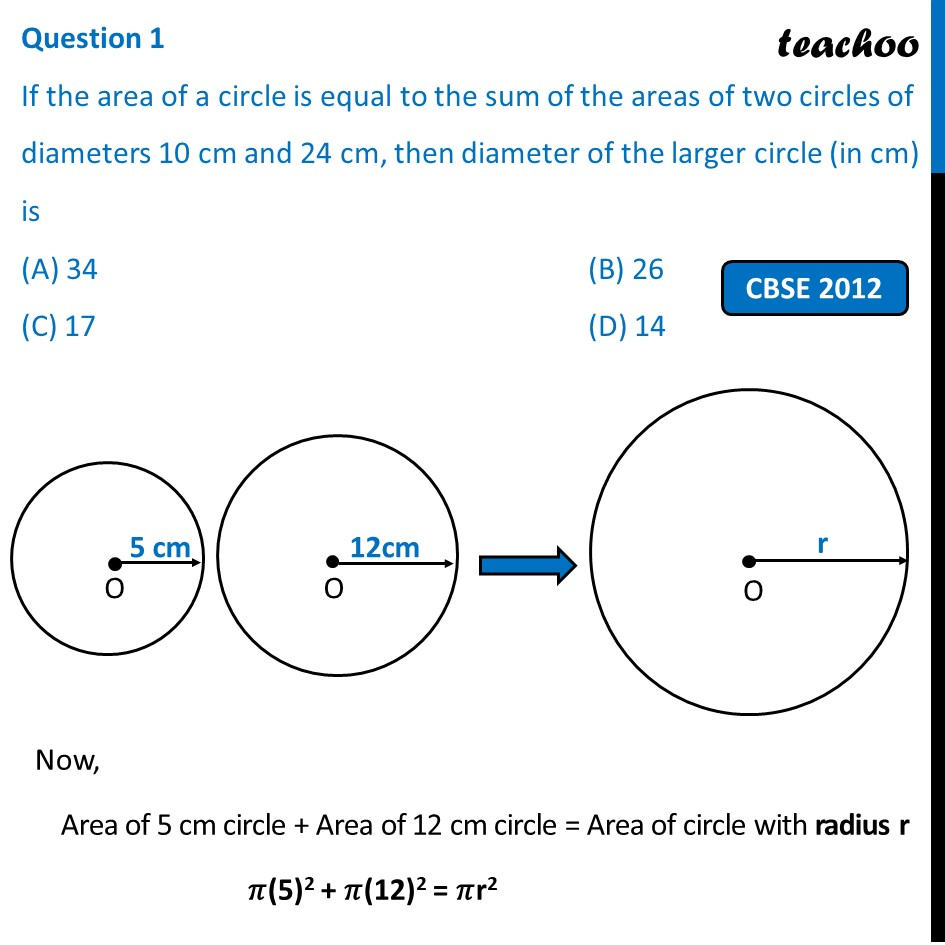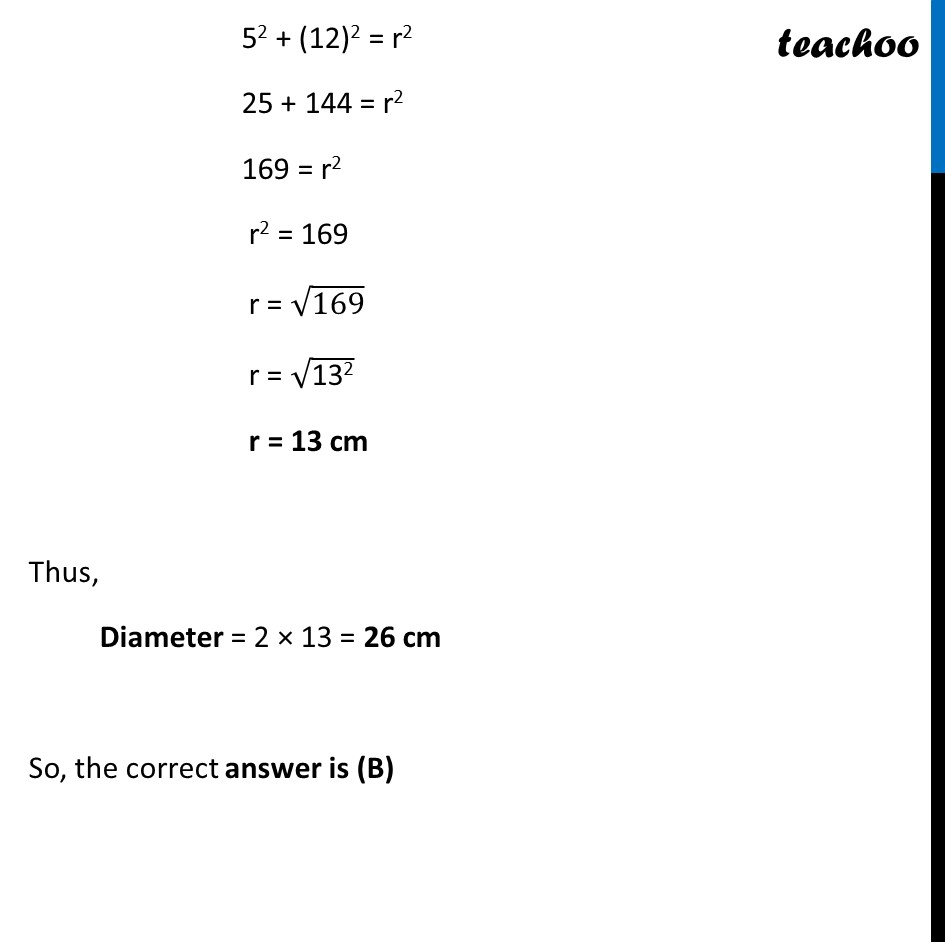Past Year MCQ

Chapter 11 Class 10 Areas related to Circles
Serial order wise

## (D) 14

This question is similar to Question 12 - NCERT Exemplar - MCQ - Chapter 12 Class 10 Areas related to CirclesLearn in your speed, with individual attention - Teachoo Maths 1-on-1 Class

### Transcript

Question 1 If the area of a circle is equal to the sum of the areas of two circles of diameters 10 cm and 24 cm, then diameter of the larger circle (in cm) is (A) 34 (B) 26 (C) 17 (D) 14 Now, Area of 5 cm circle + Area of 12 cm circle = Area of circle with radius r 𝜋(5)2 + 𝜋(12)2 = 𝜋r2 52 + (12)2 = r2 25 + 144 = r2 169 = r2 r2 = 169 r = √169 r = √("132" ) r = 13 cm Thus, Diameter = 2 × 13 = 26 cm So, the correct answer is (B)